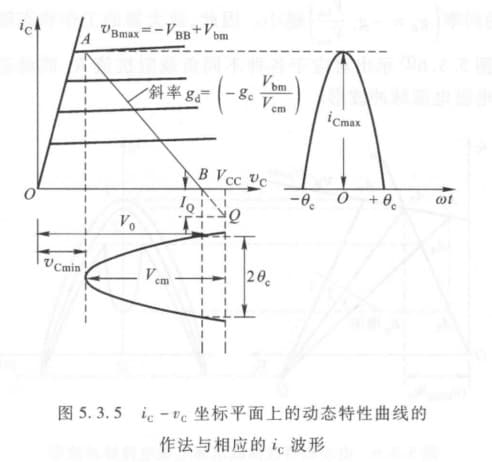# 谐振功率放大器的工作原理

1. 低频区 $f<0.5f_\beta$：不考虑电抗分量与载流子渡越时间
2. 中频区 $0.5f_\beta<f<0.2f_T$：考虑结电容
3. 高频区 $0.2f_T<f<\beta<f_T$：考虑电极引线的电感作用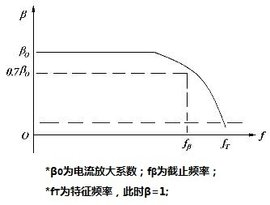## 获得高效率

1. $P_=$：直流电源供给的直流功率
2. $P_o$：交流输出信号功率
3. $P_c$：集电极耗散功率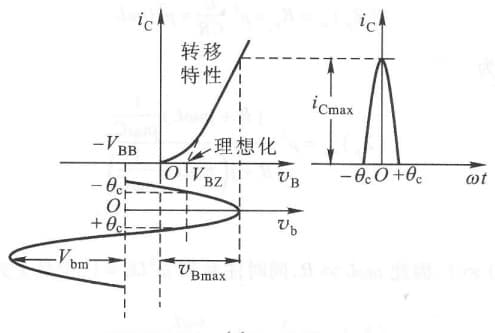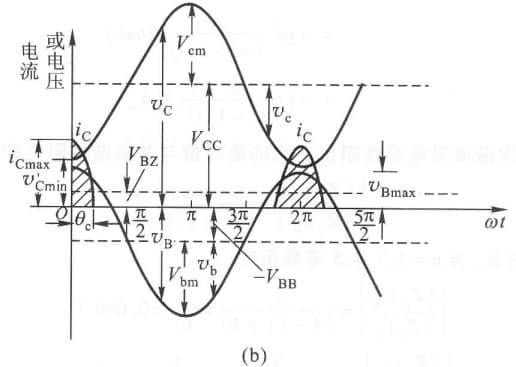• $V_{CC}$ 集电极直流电源电压
• $V_{BB}$ 基级直流偏压
• $v_c$ 集电极交流输出电压
• $v_b$ 基级交流信号
• $v_C$ 集电极到发射级的瞬时电压
• $v_B$ 基级到发射级的瞬时电压
• $i_C$ 集电极瞬时电流
• $i_B$ 基级瞬时电流
• $i_E$ 基级瞬时电流
• $2\theta_c$ 集电极流通角

# 折线近似分析法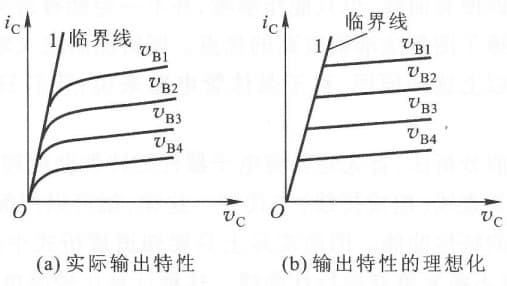1. 跨导：$g_c=\frac{\Delta i_C}{\Delta v_B} \Big\vert_{v_C为常数}$
2. 理想特性：$i_C=g_c(v_B-V_{BZ})$ （适用于 $v_B>V_{BZ}$）## 动态特性与负载特性